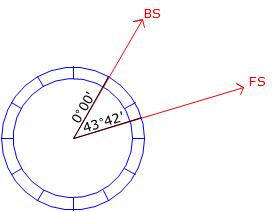## 1. Before We Start...

### a. Angle Sets

Angles are generally measured as direct and reverse (D/R) sets. How many sets are needed and how close the direct and reverse angles must be to each other depends on a number of things: survey purpose, TSI resolution, sight lengths, etc. 2. Incrementing the Circle

### b. Advancing the Circle

When measuring a horizontal angle with a repeating theodolite or transit, the horizontal circle was advanced so different parts of it would be used for the measurements. This would help compensate for circle irregularities such as axes eccentricity and division spacing irregularities. The most common method was to bring the initial FS angle back to the BS to serve as the beginning of the next measurement, Figure C-1. The angular value accumulated with successive measurements.(a) First measurement(b) Second measurement(c) Third measurement Figure C-1 Advancing the Circle

An upper and lower horizontal lock and slow motion was needed to accomplish this. Each would be used at different points in the measurement to either hold the circle or allow it to rotate.

TSIs generally have only a single lock and slow motion for the horizontal circle. The circle itself is electronically encoded so zero can be anywhere the operator chooses. Because the display is digital, the operation can also freeze or hold the reading so it doesn't change as the TSI is rotated. Manipulation of the lock and holding the reading allows the angle to accumulate also on a TSI. We'll use this method to simulate circle advancement.